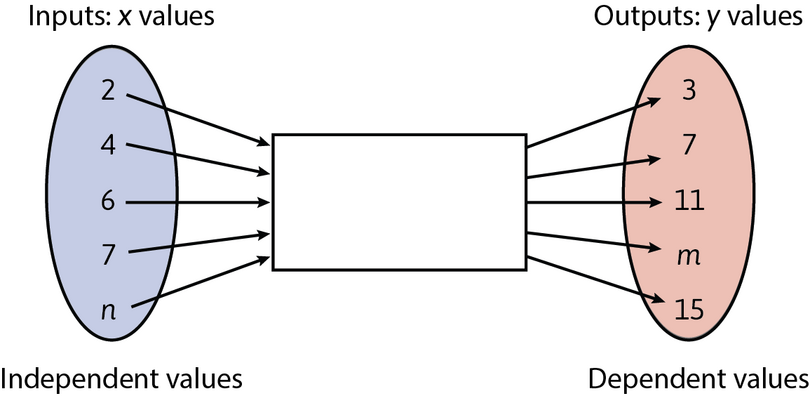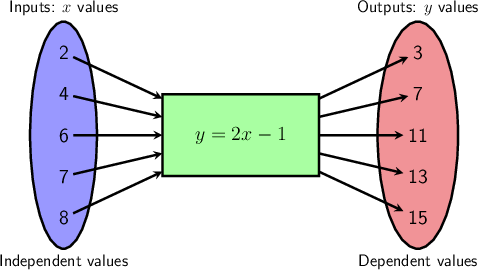Home Practice
For learners and parents For teachers and schools
Textbooks
Full catalogue
Pricing SupportLog in

We think you are located in United States. Is this correct?

# Finding the rule for flow diagrams and tables

## 5.5 Finding the rule for flow diagrams and tables

Sometimes we have information summarised and represented in a table or a flow diagram, but the rule is not given. However, by looking at the numbers in the top row and corresponding numbers in the bottom row, we can find the rule.

In some cases, more than one rule might be possible to describe the relationship between $$x$$- and $$y$$-values. Any rule is acceptable if it matches all the given input values to all the corresponding output values.

## Worked Example 5.10: Finding the rule from a flow diagram

Find the rule for calculating the output value for every given input value in the flow diagram below. Use the rule to find the missing values of $$m$$ and $$n$$.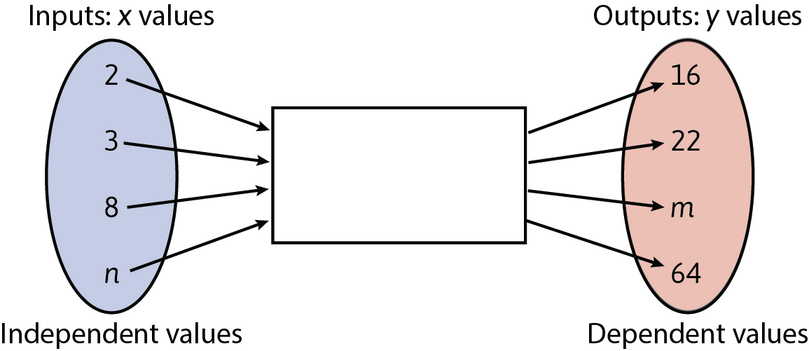### Find the relationship between the input and output numbers in the flow diagram.

Use the method of trial-and-error to find the rule. We only have two complete ordered pairs: $$(2; 16)$$ and $$(3; 22)$$.

\begin{align} 2 \times 6 + 4 &= 16 \\ 3 \times 6 + 4 &= 22 \end{align}

So, the rule could be: “multiply by $$6$$ and add $$4$$”.

The method of trial-and-error is a way of finding a solution by making varied attempts until the answer is found.

In this example, we have two complete ordered pairs: $$(2;16)$$ and $$(3;22)$$, so we need to look at different ways to get from the first to the second number:

$$2 \times 8 = 16$$, but $$3 \times 8 = 24 \neq 22$$, so the rule is not “multiply by $$8$$”.

$$2 \times 5 + 6 = 16$$, but $$3 \times 5 + 6 = 21 \neq 22$$, so the rule is not “multiply by $$5$$ and add $$6$$”.

Keep trying!

### Write the rule.

Translate the discovered relationship into a mathematical formula:

\begin{align} \text{input } \times 6 + 4 &= \text{ output} \\ x \times 6 + 4 &= y \end{align}

So, $$y = 6x + 4$$ describes the relationship between the $$x$$ values and the given $$y$$ values.

### Use the rule to find the missing value, $$m$$.

If $$x = 8$$, then:

\begin{align} y &= 6(8) + 4 \\ &= 48 + 4 \\ &= 52 \end{align}

So, $$m = 52$$.

### Use the rule to find the missing value, $$n$$.

If $$y = 64$$, then $$64 = 6(x) + 4$$.

Ask yourself, “What do I need to multiply $$6$$ by to get close to $$64$$?” ($$64$$ is the value on the other side of the equal sign.)

If you multiply by $$10$$, then $$6 \times 10 = 60$$.

Now add $$4$$:

$6(10) + 4 = 64$

So, if $$y = 64$$, then $$x = 10$$.

$$n$$ is an input value, so it is the same as this $$x$$-value.

So, $$n = 10$$.

The completed flow diagram looks like this: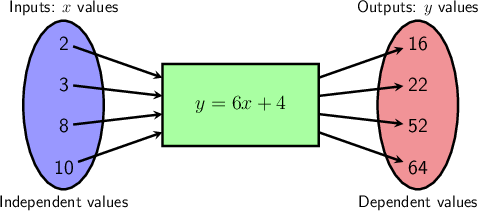## Worked Example 5.11: Finding the rule from a table of values

Describe the relationship between the numbers in the top and bottom rows of the table. Then write down the values of $$m$$ and $$n$$.

 $$x$$ $$–2$$ $$–1$$ $$0$$ $$1$$ $$2$$ $$12$$ $$n$$ $$y$$ $$–7$$ $$–5$$ $$–3$$ $$–1$$ $$1$$ $$m$$ $$35$$

### Find the relationship between top and bottom numbers in the table.

Looking at the first three input values and their corresponding output values, we can find that the numbers in the top row are doubled and then reduced by $$3$$.

\begin{alignat}{3} -2 \times 2 - 3 =& -4 - 3 &= -7 \\ -1 \times 2 - 3 =& -2 - 3 &= -5 \\ 0 \times 2 - 3 = & 0 - 3 &= -3 \end{alignat}

### Write the rule.

Translate the discovered relationship into mathematical formula:

\begin{align} \text{input } \times 2 - 3 &= \text{ output} \\ x \times 2 - 3 &= y \end{align}

So, $$y = 2x - 3$$ describes the relationship between the $$x$$-values and the given $$y$$-values.

### Use the rule to find the missing value of $$m$$.

To find $$m$$, we have to place the corresponding values for $$x$$ in the rule and work out the output value for $$y$$.

If $$x = 12$$, then:

\begin{align} y &= 2 \times 12 - 3 \\ &= 24 - 3 \\ &= 21 \end{align}

So, $$m = 21$$.

### Use the rule to find the missing value of $$n$$.

To find $$n$$, we have to place the corresponding value for $$y$$ in the rule and solve the equation by inspection.

If $$y = 35$$, then $$35 = 2x - 3$$.

When solving the equation by inspection, we are looking for a number such that when multiplied by $$2$$, is $$3$$ more than $$35$$: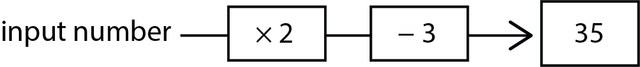\begin{align} 19 \times 2 &= 38 \\ 38 - 3 &= 35 \end{align} \begin{align} 35 &= 2x - 3 \\ &= 2(19) - 3 \end{align}

So, $$n = 19$$.

# Test yourself now

High marks in science are the key to your success and future plans. Test yourself and learn more on Siyavula Practice.

Exercise 5.1: Complete flow diagrams and tables

Describe the relationship between the numbers in the top and bottom rows in the table. Then write down the values of $$m$$ and $$n$$.

 $$x$$ $$–2$$ $$–1$$ $$0$$ $$1$$ $$2$$ … $$6$$ $$n$$ $$y$$ $$4$$ $$1$$ $$0$$ $$1$$ $$4$$ … $$m$$ $$81$$

$$y = x^{2}$$; $$m = 36$$; $$n = 9$$

Describe the relationship between the numbers in the top and bottom rows in the table. Then write down the values of $$m$$ and $$n$$.

 $$x$$ $$–2$$ $$–1$$ $$0$$ $$1$$ $$2$$ … $$100$$ $$n$$ $$y$$ $$8$$ $$9$$ $$10$$ $$11$$ $$12$$ … $$m$$ $$210$$

$$y = x + 10$$; $$m = 110$$; $$n = 200$$

Describe the relationship between the numbers in the top and bottom rows in the table. Then write down the values of $$m$$ and $$n$$.

 $$x$$ $$–3$$ $$–2$$ $$0$$ $$2$$ $$3$$ … $$10$$ $$n$$ $$y$$ $$7$$ $$2$$ $$–2$$ $$2$$ $$7$$ … $$m$$ $$398$$

$$y = x^{2} - 2$$; $$m = 98$$; $$n = 20$$

Find the rule for calculating the output value for every given input value in the flow diagram below.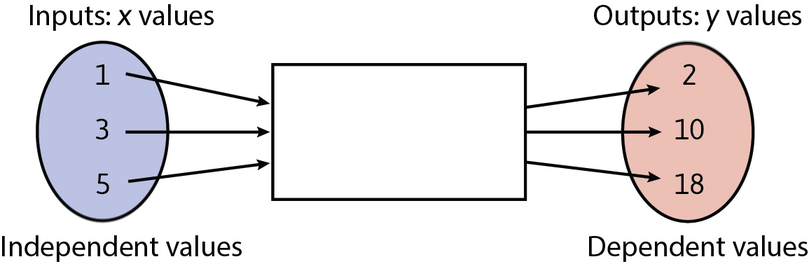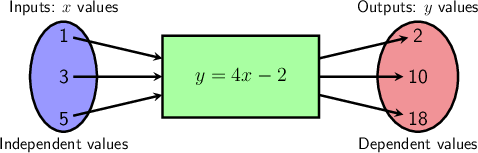Find the rule for calculating the output value for every given input value in the flow diagram below.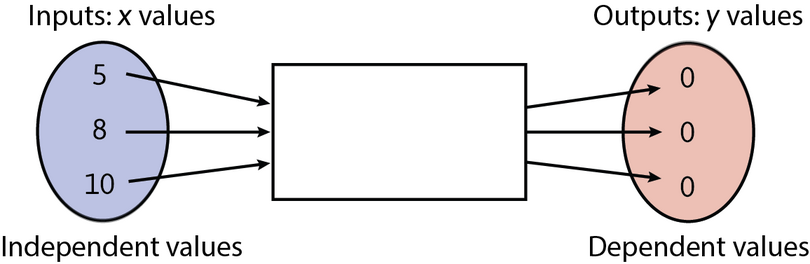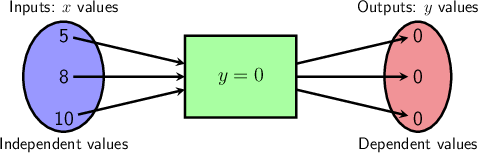Find the rule for calculating the output value for every given input value in the flow diagram below. Use the rule to calculate the values of $$m$$ and $$n$$.